Maurer-Cartan form

Let$G$ be a (connected) Lie group. The Maurer-Cartan form of$G$ is a 1-form on$G$ with values in the Lie algebra$\mathfrak{g}$, given as follows.
The tangent vector$v$ at a point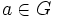$a \in G$, is sent to the unique vector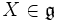$X \in \mathfrak{g}$ which is mapped by the differential of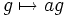$g \mapsto ag$, to$v$. In other words, the map is: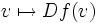$v \mapsto Df(v)$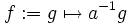$f := g \mapsto a^{-1}g$